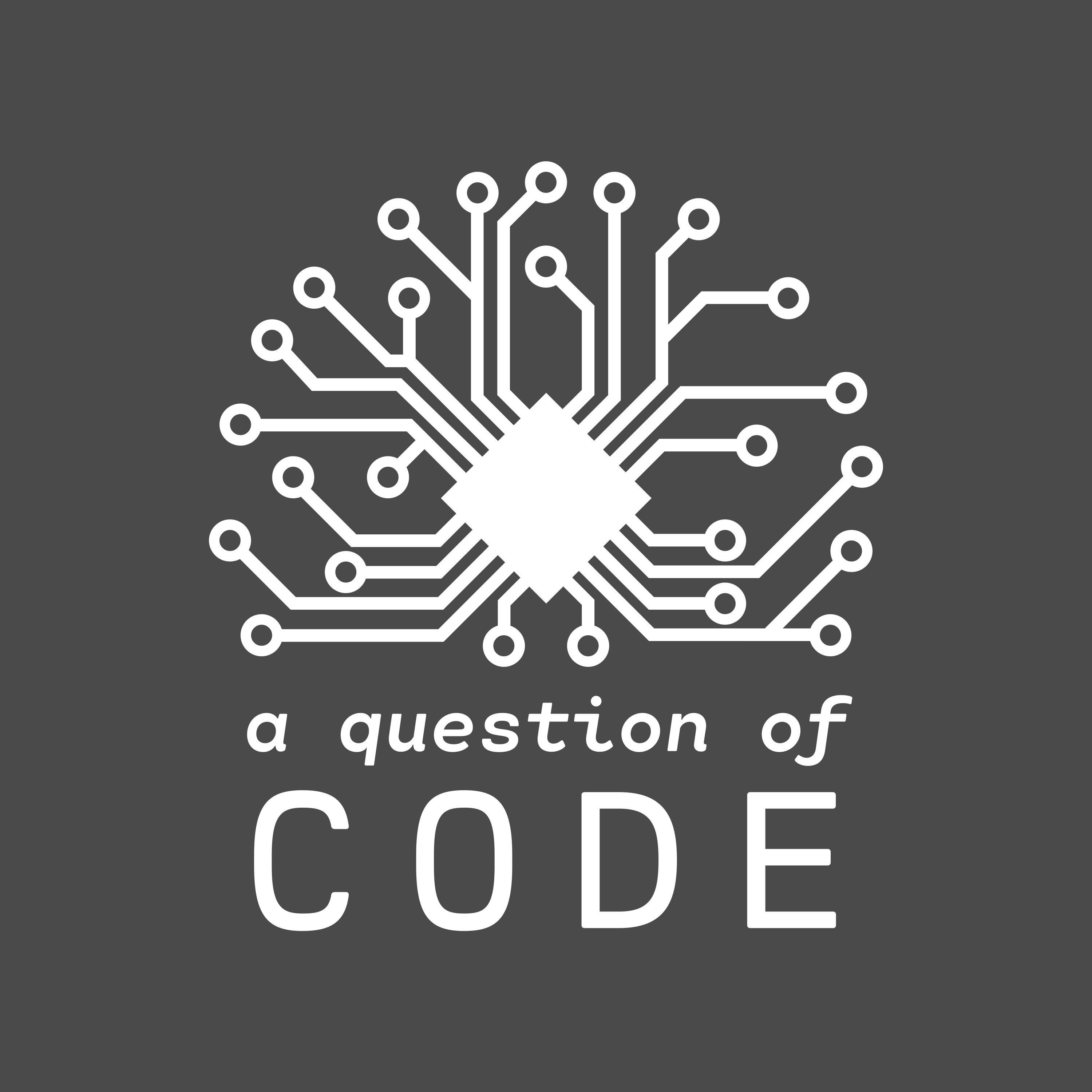# Do you need to be good at maths to become a programmer?

Episode #25:
Modified onIt's a common assumption that all programmers are maths wünderkinds. But is that necessarily the case? Many coders do come have a strong mathematical background, but does the correlation imply causation? What is like to learn to code when you've previously been a humanities specialist? Can you get by without any maths at all? Find out all this, and more, in this week's illogical instalment of A Question of Code.

## Useful bits of maths:

1. Calculus (used in neural networks and "A.I.")
2. Linear algebra (used for calculating 3D geometry, specifically helpful when transforming matrices)
3. Geometry and trigonometry (used for calculating positions for graphs and data-visualisations)
4. Lambda calculus (foundational part of Functional Programming)